×

Search anything:

# Different Pivot selection in Quick Sort

#### Sorting Algorithms quick sort AlgorithmsIn this article, we have explored Different Pivot selection techniques in Quick Sort such as Median of Medians, Mode, First Element and much more along with Time Complexity of all methods.

1. Introduction to Quick Sort
2. Pivot Selection
3. Different Pivot Selection Techniques

## Introduction to Quick Sort

Quicksort has been described as the "quickest" and the "most efficient" sorting algorithm present.

Quicksort is a sorting algorithm based on the divide and conquer technique i.e., it breaks down a problem into smaller subproblems.

Quicksort sorts a collection of elements by selecting a pivot point and partitioning the collection around it, with components smaller than the pivot appearing before the pivot element and the larger ones appearing after the pivot element. It continues to select a pivot point and break down the collection until single element lists are obtained which are then combined to form a single sorted list.

### Algorithm of Quicksort

1. A pivot element is determined which is usually an arbitrary element
2. Next, the algorithm uses partitioning logic to partition the large collection of elements into smaller collection of elements in such a way that the elements smaller than the pivot are moved towards the left of pivot and the elements greater than the pivot are moved to the right of pivot.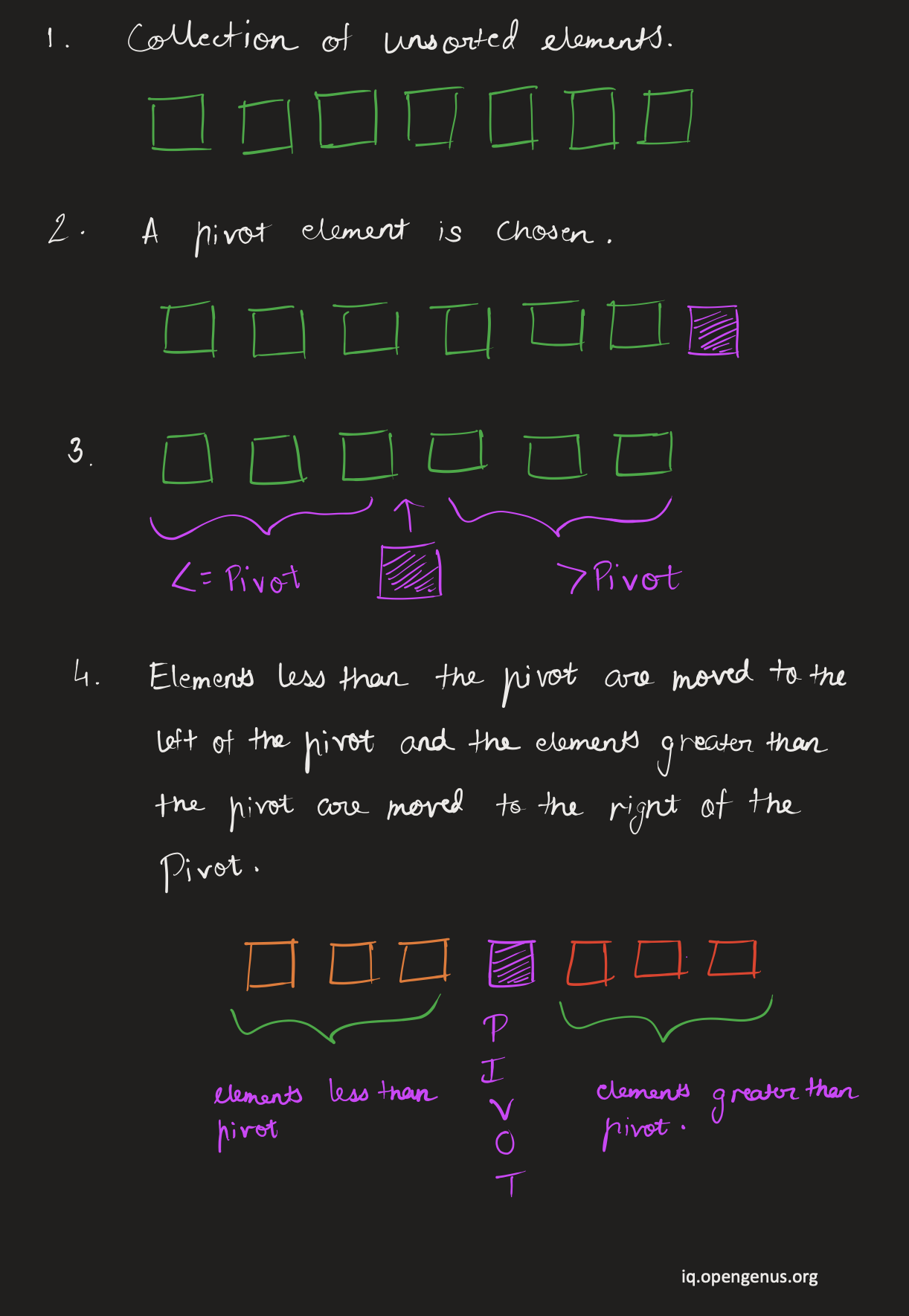1. After the larger collection is divided into small collections of elements, the quicksort algorithm recursively uses the same process of choosing a pivot and partitioning the collection on the smaller collection of elements until single element collections are obtained.
2. The single element collection are then merged together to result in a sorted array.

We know the items are in the correct order in relation to the pivot even though the collection isn't completely sorted yet. This indicates that components on the left side of the partition will never be compared to elements on the right side of the partition. We already know they're positioned properly in reference to the pivot.

### Working of Quicksort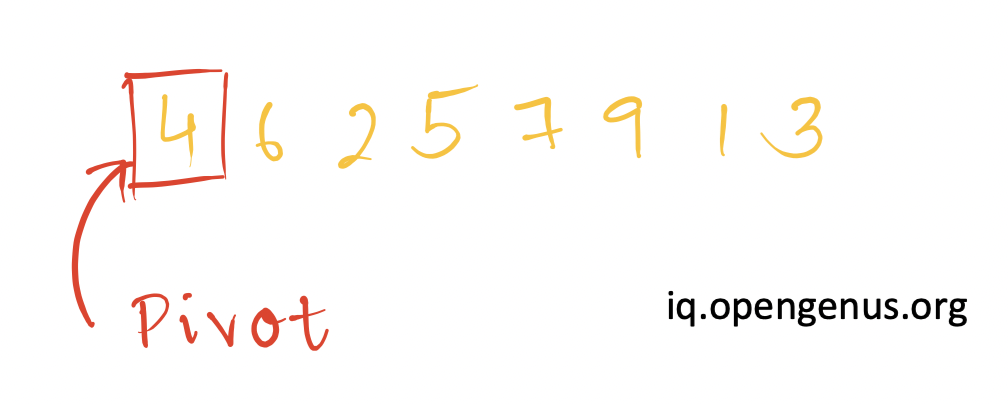We begin by considering the first element as the pivot from a given collection of elements.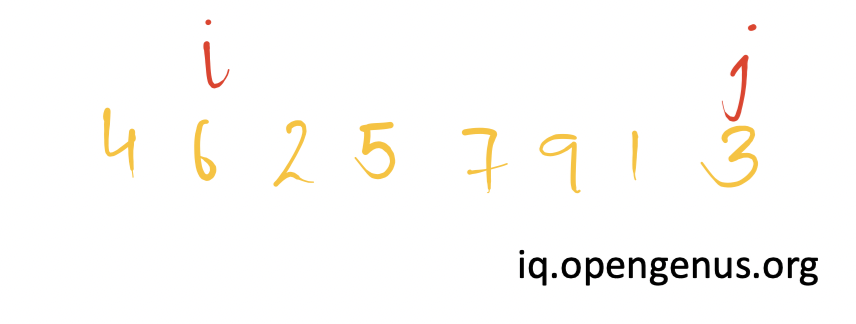Then, we consider two reference pointer i and j. i points to element immediately after the pivot and j points to last element.
Since 6>pivot and 3<pivot, the reference pointers are not moved any further.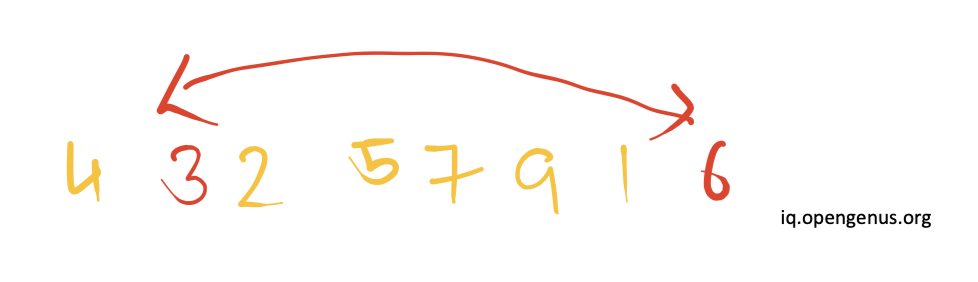The elements present at the position of i and j are swapped with each other.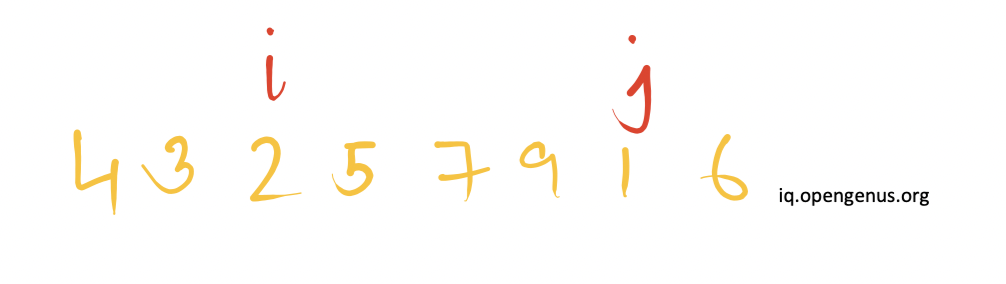Next, the reference pointer i is incremented(moved to right) and the pointer j is decremented(moved to left). Since 2<pivot and j<pivot, therefore the reference pointer i will be incremented further whereas there will be no change in the position of reference pointer j.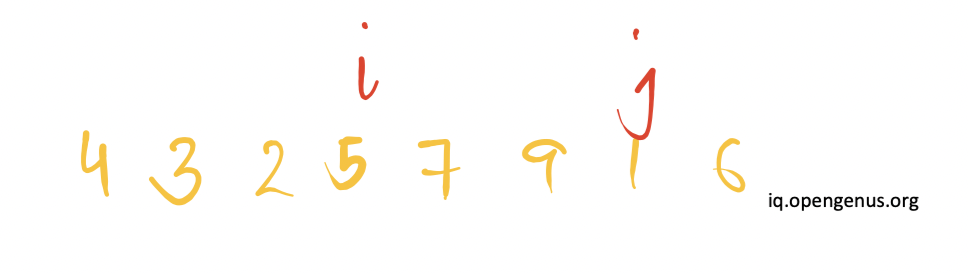Now 5>pivot hence there will be no change in the position of reference pointers.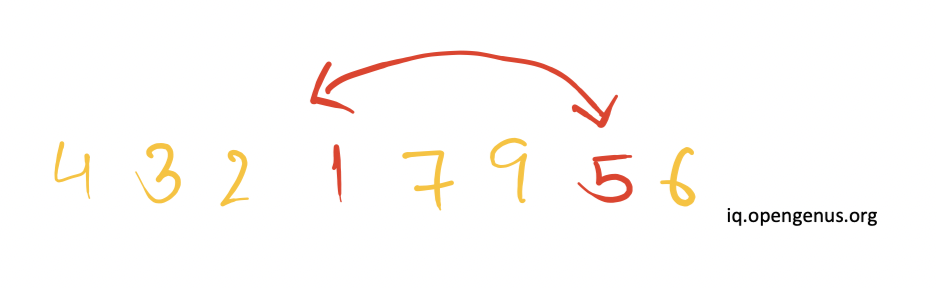The elements present at the position of i and j are swapped with each other.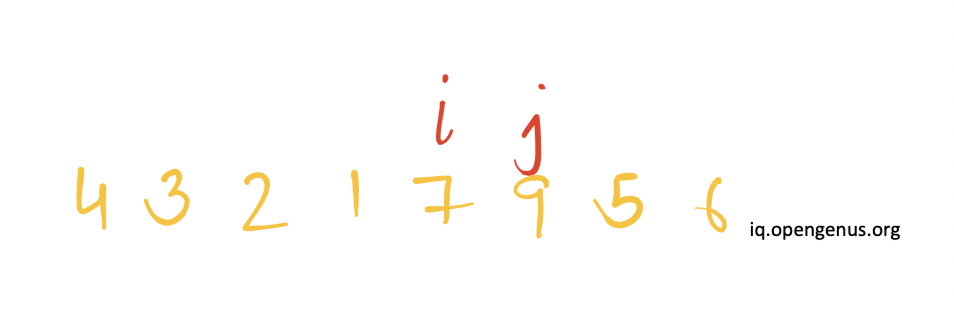Next, the reference pointer i is incremented(moved to right) and the pointer j is decremented(moved to left).Since 7>pivot and 9>pivot, therefore the reference pointer j will be decremented further whereas there will be no change in the position of reference pointer i.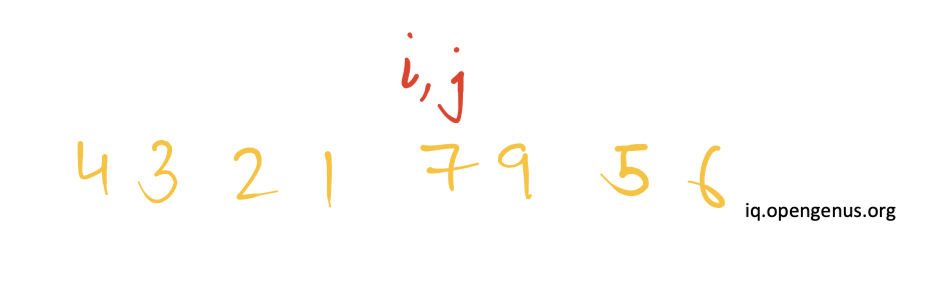Now 7>pivot hence the reference pointer j will be decremented further.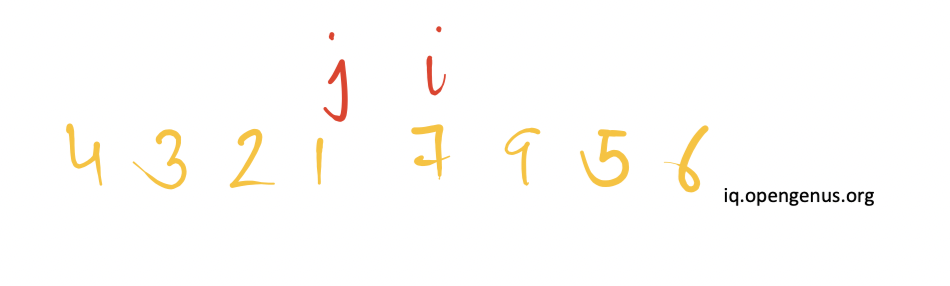Now 1<pivot hence, there will be no change in the position of reference pointers.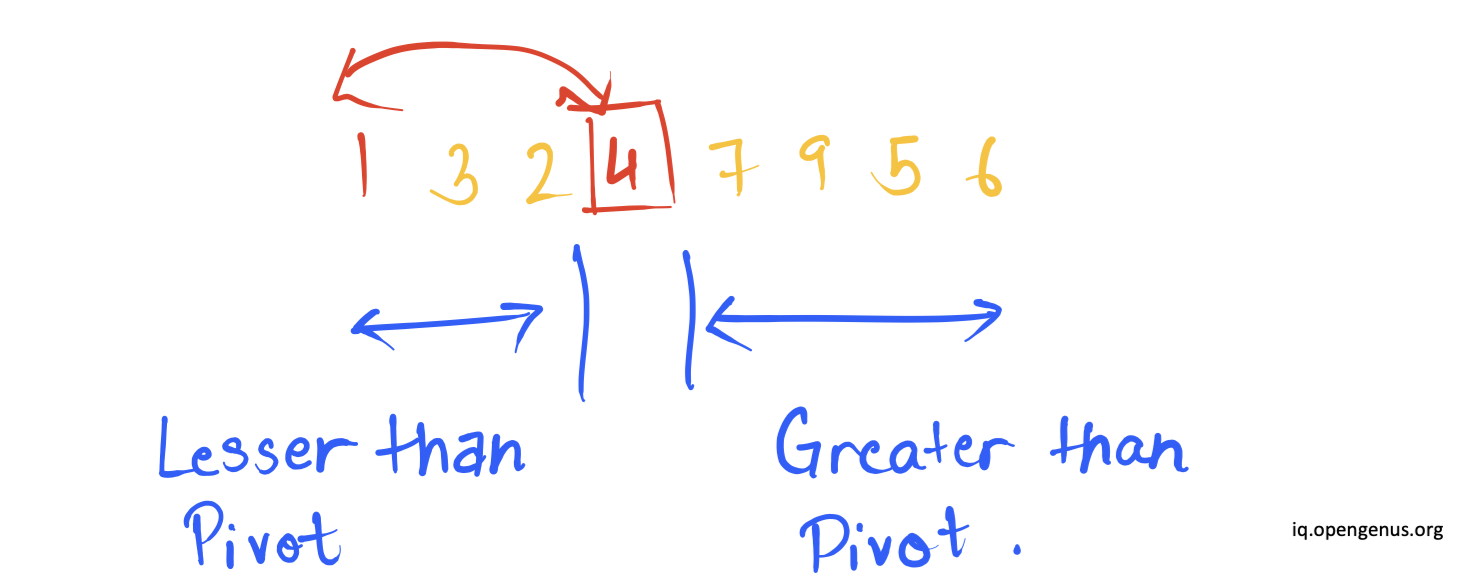Now position of pointer i>position of pointer j therefore, the element at the pivot position will be swapped with the element present at the position of reference pointer j.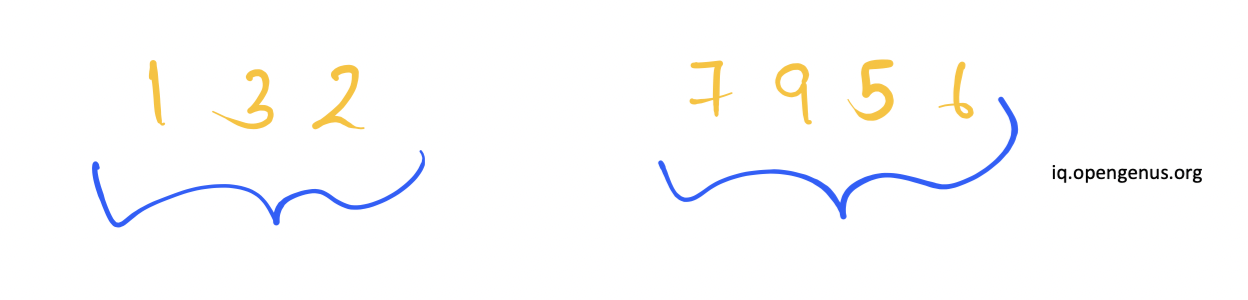We see that the collection is now divided into two parts where the entire list isn't sorted but elements are in sorted with respect to the pivot and the pivot element is at its correct position in the sorted order.

The same algorithm is now applied recursively to each of the partitioned collection until single element collections are obtained.

## Pivot Selection

There isn't any right or wrong choice for pivot element, per se. However the choice of pivot element turns out be crucial for the performance of quicksort algorithm, varying its time complexity from upto twice as fast from its competitors (O(n logn)) to a worst case with time complexity O(n^2).

Ideally, we want the elements smaller than the pivot and the elements larger than the pivot to be mostly equal in the two partitioned portions. We may encounter the worst case scenario if they are unequal or imbalanced.

In actuality, there are various approaches to make the worst scenario for a quicksort algorithm exceptionally uncommon. Instead of the first element or the last element in the list, another fixed element, such as the middle element, could be used as the partitioning element. While this can help in some cases, basic oddities can still happen. Using a random dividing element virtually eliminates the occurrence of anomalous cases in practical situations.

## Choosing a Good Pivot

The optimal pivot would be the collection's median; however, this is difficult to calculate and would significantly slow down the method.
Various methods for obtaining medians actually increase the algorithm's average performance while also making the worst case scenario unlikely to occur in practice.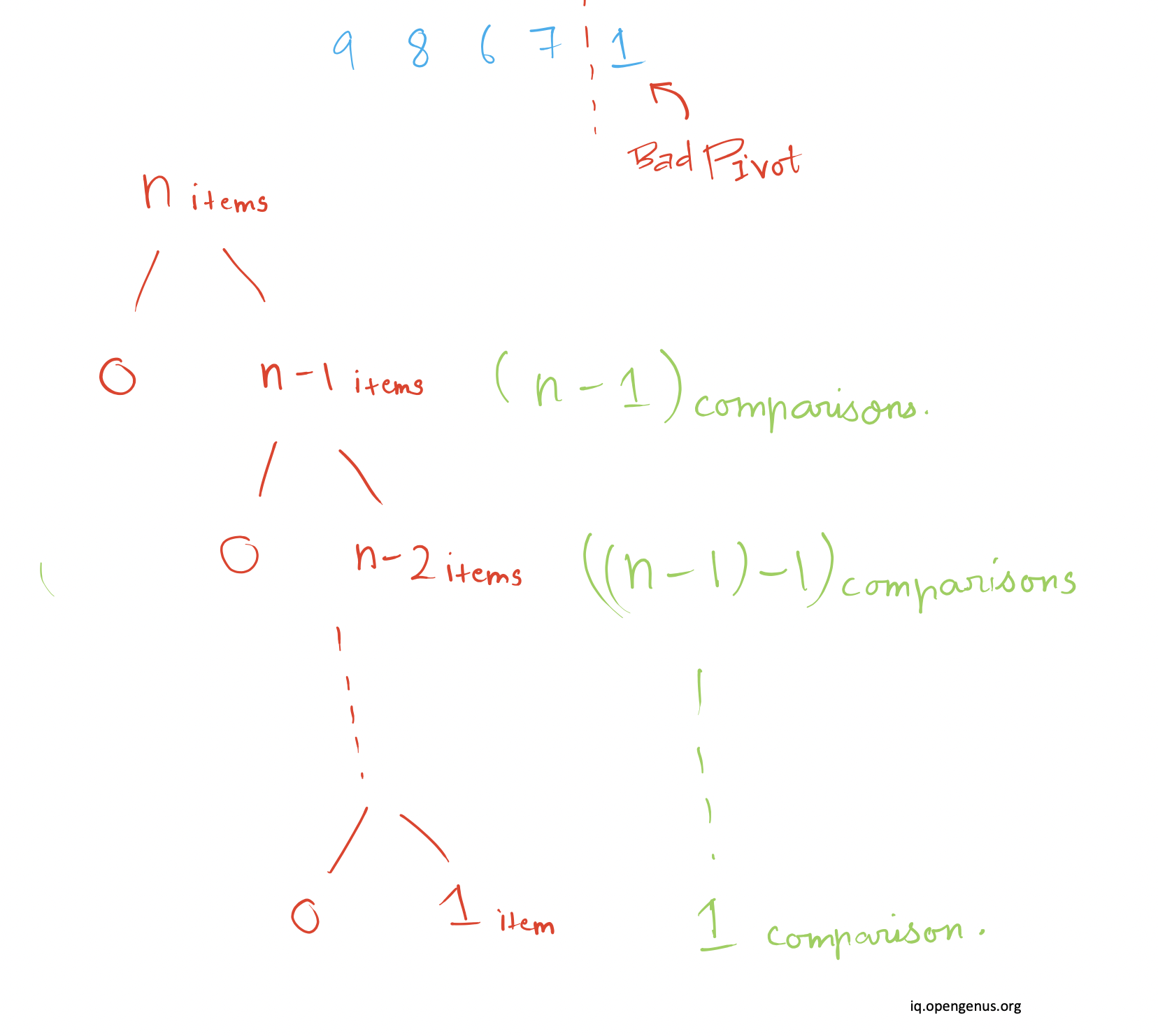Choosing a bad pivot results from either choosing the largest item of the collection or the smallest item of the collection.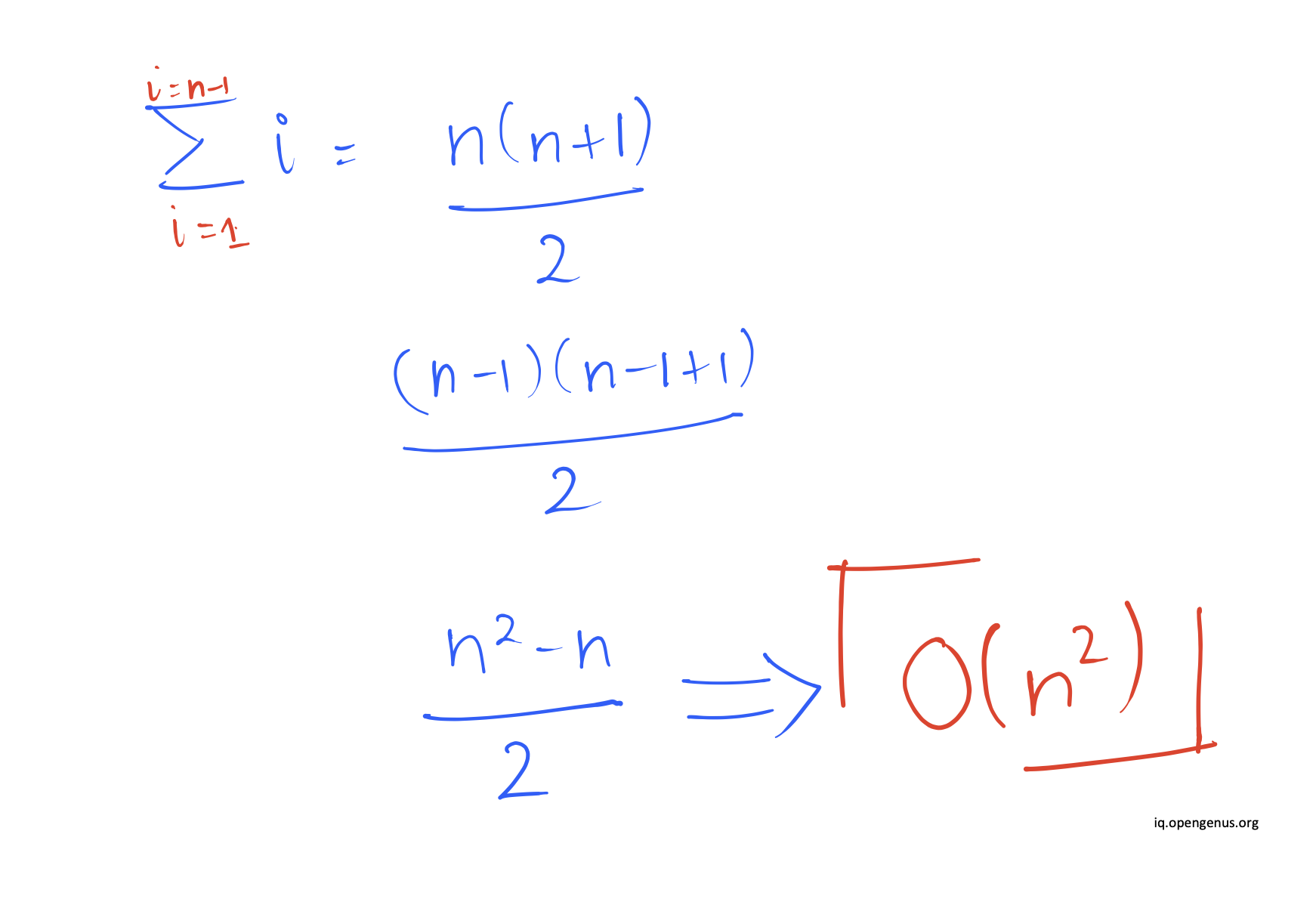As a result, the splitting into subcollections occurs at either index 0 (beginning) or index n (end). This divides an n-item collection into 1 item less than the previous level and 0 items.

This method of selecting a "bad pivot" and then partitioning n-1 and 0 is performed recursively, resulting in an extremely skewed tree and, in the worst case, a quicksort algorithm that runs in O(n^2).

## Different Pivot Selection Techniques

The different types of Pivot Selection in Quick Sort are as follows:

• Median
• Median-of-three method
• Median-of-five with random index
• Median-of-five without random index
• Mean as Pivot
• Mode as Pivot
• i-th Element as Pivot
• Largest/ Smallest Element

## Median as Pivot

Finding the actual median of an array is a challenging problem. In practice, it will take O(N logN) time to find the median as it is same as sorting the data. In theory, it can be done in O(N) but the constant factors are high. So, finding the true median and using to as the pivot is not feasible.

The alternative is to use algorithms that give a good estimate of the median that is an element that is closer to the median.

### Median-of-three method for Pivot in Quick Sort

At each stage of this approach, samples of size three are employed. Primarily, sampling ensures that the dividing components do not always appear at the bottom of the sub lists. The approach employs the first, middle, and last components as samples and the median of those three as the dividing element to make the worst scenario improbable. Using this strategy, the worst case for sorted input is clearly eliminated, and quicksort's running time is reduced by around 5%.

### Median-of-five with random index selection method

The method employs a sample size of 5 components, including the first, middle, and last elements, as well as two more elements chosen at random between the first and last elements using a random number generating function. Although this strategy improves load balancing and cuts quicksort execution time by more than 5%, there is a cost associated with random number creation.

### Median-of-five without random index selection method

This method, like the median-of-five with random index selection scheme, uses a sample size of five items. The five elements are chosen in the following order: first, middle, last, and two others at positions [(low+high)/4] and [3*(low+high)/4], where low and high are the indexes of the first and last entries in the original array, respectively. As a result, it creates a new five-element array that the algorithm will employ. After sorting the new array, it returns the middle member. The partitioning element is the returned middle element, which is the median of those five elements. As a result, the usage of random index selection is no longer necessary.

• Worst Case Time complexity of Quick Sort with the above the median algorithms: O(N logN)
• Worst Case Time Complexity of Quick Sort with true median: O(N logN logN)

## Mean as Pivot

When the collection is sorted, choosing the pivot as the mean of the collection results in the best case running time for the quick sort algorithm because the mean element is close to or equal to the median element, which divides our collection into equal halves in each recursion, whereas choosing the mean of an unsorted collection of elements gives a mean, which when chosen as the pivot element will partition our collection into skewed parts consisting of 1 and N-1 elements.

• Best Case Time Complexity:O(N logN)
• Worst Case Time Complexity:O(N^2)

## Mode as Pivot

When the collection's mode is chosen as the pivot element, the algorithm's running time will be best case time complexity only when the mode is an element close to or equal to the collection's median, because our collection will be partitioned into two equal halves, whereas the mode being closer to the greatest or smallest element will result in a skewed partition and a worst case time complexity of O(n^2).

• Best Case Time Complexity: O(N logN)
• Worst Case Time Complexity: O(N^2)

## i-th Element as Pivot

When the pivot element for the algorithm is picked at random, it is assumed that the element chosen will be near to the collection's median, resulting in a pivot partitioning the collection into two equal halves and a best case time complexity. However, the largest/smallest element, or an element near to the largest/smallest, could be chosen as the pivot, resulting in a pivot splitting the collection in a skewed manner and, as a result, a worst-case time complexity.

• Best Case Time Complexity: O(N logN)
• Worst Case Time Complexity: O(N^2)

## Largest/ Smallest Element

Choosing the largest/smallest element as the pivot results in the worst case time complexity for the algorithm because the splitting of the collection occurs at either index 0 (beginning) or index n (end) which divides an n-item collection into 1 and n-1 items recursively as the algorithm proceeds.

This results in an extremely skewed tree and results in a quicksort running in O(n^2).

• Best Case Time Complexity: O(N logN)
• Worst Case Time Complexity: O(N^2)

With this article at OpenGenus, you must have the complete idea of different Pivots that can be used with Quick Sort.